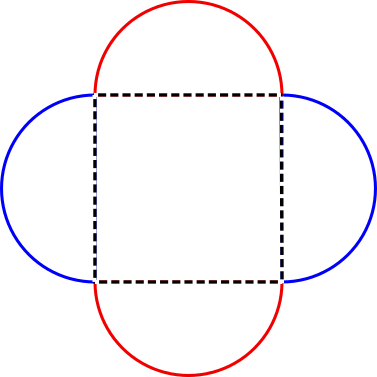#### You may also like### Building Tetrahedra

Can you make a tetrahedron whose faces all have the same perimeter?A 1 metre cube has one face on the ground and one face against a wall. A 4 metre ladder leans against the wall and just touches the cube. How high is the top of the ladder above the ground?Four rods are hinged at their ends to form a convex quadrilateral. Investigate the different shapes that the quadrilateral can take. Be patient this problem may be slow to load.

# Bobbly Perimeter

##### Age 14 to 16 ShortChallenge Level
Finding the lengths of the arcs
If the perimeter of the square is 20 cm, then each side must be 5 cm long.

The sides of the square are the diameters of the semicircles, so the circumferences of the full circles would be $5\times\pi=5\pi$ cm.

As shown in the diagram below, the 4 semicircles make up 2 full circles.So the total perimeter is $5\pi+5\pi=10\pi$ cm.

Using scale factors
The sides of the square are the diameters of the semicircles, and so the circumferences of the full circles would be $\pi\times\text{diameter}=\pi\times\text{side length}$.

Each semicircle has only half the circumference of a full circle, so its length is $\frac{1}{2}\pi\times\text{side length}$.

So to go from a square to a semicircle, each side length is mutliplied by a scale factor of $\frac{1}{2}\pi$. So the perimeter must also be multiplied by this scale factor. So the perimeter of the new shape will be $20\times\frac{1}{2}\pi=10\pi$ cm.

You can find more short problems, arranged by curriculum topic, in our short problems collection.